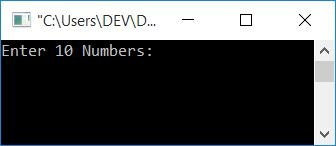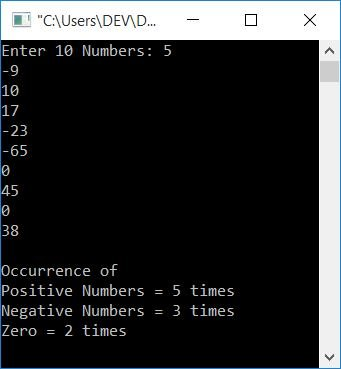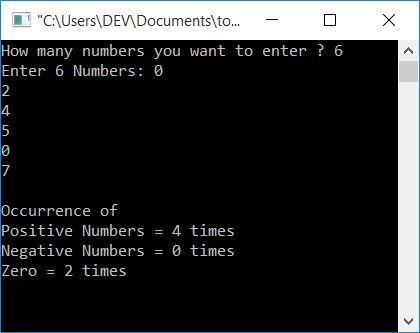# C Program to Count Positive, Zero, and Negative Numbers

In this article, you will learn and get code about counting the occurrence of positive numbers, negative numbers, and zero from the given list of numbers by the user (at run-time).

## Count the number of positive, negative, and zero occurrences in C

To count the number of positive numbers, negative numbers, and zero from the given set of numbers entered by the user in C programming, apply a loop to check all the numbers in a way that determines whether the current number is 0, less than 0, or greater than 0. and increment the corresponding variable's value as shown in the program given below.

```#include<stdio.h>
#include<conio.h>
int main()
{
int countPositive=0, countNegative=0, countZero=0, arr, i;
printf("Enter 10 Numbers: ");
for(i=0; i<10; i++)
scanf("%d", &arr[i]);
for(i=0; i<n; i++)
{
if(arr[i]<0)
countNegative++;
else if(arr[i]>0)
countPositive++;
else
countZero++;
}
printf("\nOccurrence of");
printf("\nPositive Numbers = %d times", countPositive);
printf("\nNegative Numbers = %d times", countNegative);
printf("\nZero = %d times", countZero);
getch();
return 0;
}```

This program was built and run using the Code::Blocks IDE. Here is the sample run:Supply any 10 numbers, say 5, -9, 10, 17, -23, -65, 0, 45, 0, 38, as input, and press the ENTER key to see the output as given in the following snapshot:You saw the above output because -9, -23, and -65 are 3 negative numbers, 5, 10, 17, 45, and 38 are 5 positive numbers, and 0 and 0 are two zeros from all the given 10 numbers.

### Allow the user to define the size

Here is another program that allows the user to define the size of the array (how many numbers he wants to enter). Let's have a look at the program:

```#include<stdio.h>
#include<conio.h>
int main()
{
int countPositive=0, countNegative=0, countZero=0, arr, n, i;
printf("How many numbers you want to enter ? ");
scanf("%d", &n);
printf("Enter %d Numbers: ", n);
for(i=0; i<n; i++)
scanf("%d", &arr[i]);
for(i=0; i<n; i++)
{
if(arr[i]<0)
countNegative++;
else if(arr[i]>0)
countPositive++;
else
countZero++;
}
printf("\nOccurrence of");
printf("\nPositive Numbers = %d times", countPositive);
printf("\nNegative Numbers = %d times", countNegative);
printf("\nZero = %d times", countZero);
getch();
return 0;
}```

The snapshot given below shows a sample run of the above program:#### The same program in different languages

C Quiz

« Previous Program Next Program »